# Work with different data formats (binary, netCDF, .mat)¶

Nikolay Koldunov

[email protected]

This is part of Python for Geosciences notes.

================

In :
%matplotlib inline
import matplotlib.pylab as plt
import numpy as np


## Binary data¶

### Open binary¶

In :
# !wget ftp://sidads.colorado.edu/pub/DATASETS/nsidc0051_gsfc_nasateam_seaice/final-gsfc/north/monthly/nt_200709_f13_v1.1_n.bin


Create file id:

In :
ice = np.fromfile('nt_200709_f13_v1.1_n.bin', dtype='uint8')


We use uint8 data type. List of numpy data types

The file format consists of a 300-byte descriptive header followed by a two-dimensional array.

In :
ice = ice[300:]


Reshape

In :
ice = ice.reshape(448,304)


Simple visualisation of array with imshow (Matplotlib function):

In :
plt.imshow(ice)
plt.colorbar();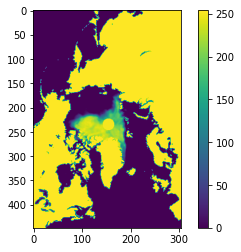To convert to the fractional parameter range of 0.0 to 1.0, divide the scaled data in the file by 250.

In :
ice = ice/250.
plt.imshow(ice)
plt.colorbar();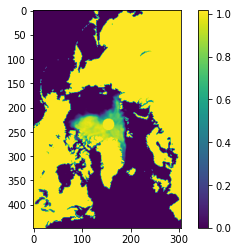Let's mask all land and missing values:

In :
plt.imshow(ice, vmin=0, vmax=1.0)
plt.colorbar();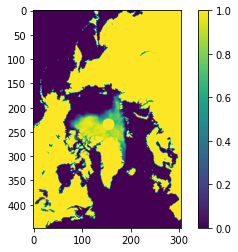In :
ice_masked = np.ma.masked_greater(ice, 1.0)
plt.colorbar();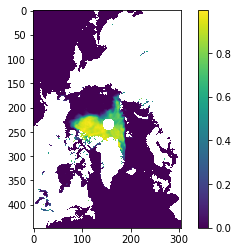### Save binary¶

In :
fid = open('My_ice_2007.bin', 'wb')
ice.tofile(fid)
fid.close()


In order to work with other data formats we need to use one of the SciPy submodules:

## SciPy¶General purpose scientific library (that consist of bunch of sublibraries) and builds on NumPy arrays.

We are going to use only scipy.io library.

## scipy.io¶

### Open .mat files¶

First we have to load function that works with Matlab files:

In :
from scipy.io import loadmat


We are going to download Polar science center Hydrographic Climatology (PHC) for January in Matlab format.

In :
# !wget https://www.dropbox.com/s/0kuzvz03gw6d393/PHC_jan.mat


Open file:

In :
all_variables = loadmat('PHC_jan.mat')


We can look at the names of variables stored in the file:

In :
all_variables.keys()

Out:
dict_keys(['__header__', '__version__', '__globals__', 'LAT', 'LON', 'DEPTH', 'PTEMP1'])

We need only PTEMP1 (3d potential temperature).

In :
temp = all_variables['PTEMP1']


Check variable's shape:

In :
temp.shape

Out:
(33, 180, 360)

Show surface level:

In :
plt.imshow(temp[0,:,:])
plt.colorbar();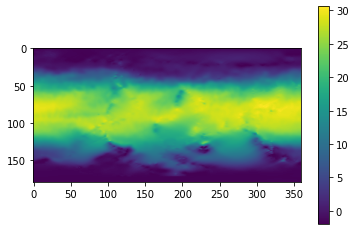### Open netCDF files¶

Scipy have function for working with netCDF files, but please don't use it it's absolete (e.g. do not support netCDF4).

The best basic option is to use python netcdf4 module that have a lot of nice functionality. Moreover NCEP reanalysis data, that we are going to work with are in netCDF4 format.

Later in the course we will also have a look at xarray that is the most convinient option nowadays.

Import nessesary function:

In :
from netCDF4 import Dataset


I am going to download NCEP reanalysis data. Surface 4 daily air temperature for 2012.

In :
# !wget ftp://ftp.cdc.noaa.gov/Datasets/ncep.reanalysis.dailyavgs/surface_gauss/air.2m.gauss.2016.nc

#Alternative for the times of US goverment shutdowns:
#!wget http://database.rish.kyoto-u.ac.jp/arch/ncep/data/ncep.reanalysis/surface/air.sig995.2012.nc


Create file id:

In :
fnc = Dataset('air.2m.gauss.2016.nc')


It's not really file id, it's netcdf_file object, that have some methods and attributes:

In :
fnc.description

Out:
'Data is from NMC initialized reanalysis\n(4x/day).  It consists of T62 variables interpolated to\npressure surfaces from model (sigma) surfaces.'
In :
fnc.history

Out:
'created 2013/12 by Hoop (netCDF2.3)'

list variables

In :
fnc.variables

Out:
{'lat': <class 'netCDF4._netCDF4.Variable'>
float32 lat(lat)
units: degrees_north
actual_range: [ 88.542 -88.542]
long_name: Latitude
standard_name: latitude
axis: Y
unlimited dimensions:
current shape = (94,)
filling on, default _FillValue of 9.969209968386869e+36 used,
'lon': <class 'netCDF4._netCDF4.Variable'>
float32 lon(lon)
units: degrees_east
long_name: Longitude
actual_range: [  0.    358.125]
standard_name: longitude
axis: X
unlimited dimensions:
current shape = (192,)
filling on, default _FillValue of 9.969209968386869e+36 used,
'time': <class 'netCDF4._netCDF4.Variable'>
float64 time(time)
long_name: Time
delta_t: 0000-00-01 00:00:00
avg_period: 0000-00-01 00:00:00
standard_name: time
axis: T
units: hours since 1800-01-01 00:00:0.0
coordinate_defines: start
actual_range: [1893408. 1902168.]
unlimited dimensions: time
current shape = (366,)
filling on, default _FillValue of 9.969209968386869e+36 used,
'air': <class 'netCDF4._netCDF4.Variable'>
float32 air(time, lat, lon)
long_name: mean Daily Air temperature at 2 m
units: degK
precision: 2
least_significant_digit: 1
GRIB_id: 11
GRIB_name: TMP
var_desc: Air temperature
dataset: NCEP Reanalysis Daily Averages
level_desc: 2 m
statistic: Mean
parent_stat: Individual Obs
missing_value: -9.96921e+36
valid_range: [150. 400.]
actual_range: [177.75    317.90002]
unlimited dimensions: time
current shape = (366, 94, 192)
filling on, default _FillValue of 9.969209968386869e+36 used,
'time_bnds': <class 'netCDF4._netCDF4.Variable'>
float64 time_bnds(time, nbnds)
unlimited dimensions: time
current shape = (366, 2)
filling on, default _FillValue of 9.969209968386869e+36 used}

In :
air = fnc.variables['air']


This time we create netcdf_variable object, that contain among other things attributes of the netCDF variable as well as data themselves.

In :
air.actual_range

Out:
array([177.75   , 317.90002], dtype=float32)
In :
air.long_name

Out:
'mean Daily Air temperature at 2 m'
In :
air.units

Out:
'degK'
In :
air.shape

Out:
(366, 94, 192)

We can access the data by simply using array syntax. Here we show first time step of our data set:

In :
plt.imshow(air[0,:,:])
plt.colorbar();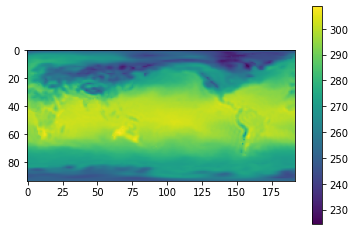## Exersise¶

• Get lat and lon variables from netCDF file
• What is the shape of this variables?
• plot 100th day of the dataset in $^{\circ}C$
In [ ]:



### Save netCDF file¶

Minimalistic variant :)

In :
!rm test_netcdf.nc
fw = Dataset('test_netcdf.nc', 'w')

fw.createDimension('t', 366)
fw.createDimension('y', 94)
fw.createDimension('x', 192)

air_var = fw.createVariable( 'air','float32', ('t', 'y', 'x'))
air_var[:] = air[:]
fw.close()


More descriptive variant:

In :
!rm test_netcdf.nc
fw = Dataset('test_netcdf.nc', 'w')

fw.createDimension('TIME', 366)
fw.createDimension('LATITUDE', 94)
fw.createDimension('LONGITUDE', 192)

time = fw.createVariable('TIME', 'f', ('TIME',))
time[:] = fnc.variables['time'][:]
time.units = 'hours since 1-1-1 00:00:0.0'

lat  = fw.createVariable('LATITUDE', 'f', ('LATITUDE',))
lat[:] = fnc.variables['lat'][:]

lon = fw.createVariable('LONGITUDE', 'f', ('LONGITUDE',))
lon[:] = fnc.variables['lon'][:]

ha = fw.createVariable('New_air','f', ('TIME', 'LATITUDE', 'LONGITUDE'))
ha[:] = air[:]
ha.missing_value = -9999.

fw.close()